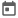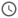# Introduction to System Verilog Data Types##### 27 Mar 2022##### 4 mins

In Verilog, all the data types were of 4-state, i.e., it could represent 0, 1, X and Z. However, in the case of test benches, these 4-state variables were not required. For example, to count the number of packets, we would require a 2-state variable. Thus, System Verilog introduces a new class of variables of 2-states, i.e., 0 and 1.

We could easily use a 4-state variable for the use case defined above. So what is the need for a 2-state variable? If we see this concerning the simulator, then to store one bit of data in a 4-state variable, the simulator would require 2 bits in memory. For more number of bits, the problem would be more severe. If we need to store only 0 and 1, there would be a significant waste of memory space. Thus, the 2-state variable was introduced to tackle this problem.

### New variables in System Verilog

In System Verilog, apart from the 2-state variable, there is a particular 4-state variable, “logic”.

#### Logic

Logic is a 4-state data type that can act both as a variable and net. In Verilog, reg can be driven in procedural blocks, and wires can be driven using the continuous assignment. Thus, it is often difficult to manage these data types, as they can be used only for a specific purpose. With the introduction of logic data type, there is a 4-state variable that can be used in both procedural and continuous assignment.

However, there are a few are limitations with the logic data type. Logic can only be used with an input and output port. If multiple drivers are driving a signal, other net data types such as wire and tri should be used. As for multiple drivers, System Verilog could determine the final state in the case of logic data type.

Example: `logic [3:0] a;`

`module xyz (inout logic data);`
`module xyz (input logic data);`
or
`module xyz (output logic data);`

#### Bit

A bit is a 2-state variable. As the name suggests, it can hold 1 bit of data at a time. Similar to other 1-bit data types like reg and wire, more than one bit can be packed together to store more than 1 bit of data.

Example: `bit [3:0] a; // It can hold 4 bits of data`.

#### Byte

A byte is also a 2-state variable, which can hold 8-bits of data. It is a signed data type, which means it can store -ve numbers in System Verilog. A byte cannot be packed together and thus will always store 8-bits of data.

Example: `byte b;`

`byte [4:0] b;` This is invalid. More details are discussed in arrays article.

#### Shortint

A shortint is a 16-bit variable having 2-states. It is also a signed data type.

#### Int

An int is similar to the integer data type in Verilog. The only difference is that int is a 2-state variable and, an integer is a 4-state variable.

Example: `int cnt = 0;`

#### Longint

A longint is a 64-bit variable having 2-state. It is also a signed data type

All the signed data types can be used as unsigned data types by using the` unsigned` keyword.

### Int Vs Integer

`int` data type is most oftenly confused with `integer` data type that we have seen in Verilog. This is why it is also a commonly asked question in interviews.

IntInteger
Available only in system VerilogAvailable in both Verilog and System Verilog
Int is 32-bit variable which is made of of bit data type.Int is a 32-bit variable which is made of reg data type
Can store only 2-state valuesCan store 4-state values
This is not synthesizableThis is synthesizable as it represents register of 32 flip-flops.
`int` data type should not be used in the RTL design as it was mainly introduced for test-bench code and also int is not synthesizable.

### Example

``````module basic_dataTypes();
// Declaring and initializing the variables
bit   [7:0] bit_data;
logic [7:0] logic_data;
reg   [7:0] reg_data;

byte signed_number;
bit [7:0] unsigned_number;

byte unsigned unsigned_byte_number;
bit signed [7:0]  signed_bit_number;

initial
begin
\$display ("Default values of data-types: \n");

\$display ("Default value of logic logic_data = %b", logic_data);
\$display ("Default value of reg reg_data     = %b", reg_data);
\$display ("Default value of bit bit_data     = %b\n", bit_data);

// initializing values

bit_data     = 8'b0101_01xz;
logic_data   = 8'b0101_01xz;
reg_data     = 8'b0101_01xz;

// Displaying the values of the variables for different bit_data types
\$display ("Initialized value for different datatypes: ");
\$display ("It may be noted that in 2-state variables x/z is treated as 0\n");

\$display ("Value of logic logic_data = %b", logic_data);
\$display ("value of reg reg_data     = %b", reg_data);
\$display ("Value of bit bit_data     = %b\n", bit_data);

\$display ("Value of integer write logic_data = %0b", integer_addr);

\$display ("Initialized value for byte and bit[7:0]:");
\$display ("It may be noted that byte will show a -ve number as it is \nby default a signed numbern\n");
signed_number   = 245;
unsigned_number = 245;
\$display ("Value of byte signed_number        = %0d", signed_number);
\$display ("Value of bit [7:0] unsigned_number = %0d\n", unsigned_number);

\$display ("It may be noted that bit [7:0] will show a -ve number as it is \ndeclared as a signed numbern\n");
signed_bit_number   = 245;
unsigned_byte_number = 245;
\$display ("Value of bit[7:0] signed_bit_number = %0d", signed_bit_number);
\$display ("Value of byte unsigned_byte_number  = %0d\n", unsigned_byte_number);
end
endmodule``````
##### Output
``````# Default values of data-types:
#
# Default value of logic logic_data = xxxxxxxx
# Default value of reg reg_data     = xxxxxxxx
# Default value of bit bit_data     = 00000000
#
# Initialized value for different datatypes:
# It may be noted that in 2-state variables x/z is treated as 0
#
# Value of logic logic_data = 010101xz
# value of reg reg_data     = 010101xz
# Value of bit bit_data     = 01010100
#
# Value of integer write logic_data = 1x101xz01xz01xz
# Value of int read logic_data      = 101010001000100
#
# Initialized value for byte and bit[7:0]:
# It may be noted that byte will show a -ve number as it is
# by default a signed numbern
#
# Value of byte signed_number        = -11
# Value of bit [7:0] unsigned_number = 245
#
# It may be noted that bit [7:0] will show a -ve number as it is
# declared as a signed numbern
#
# Value of bit[7:0] signed_bit_number = -11
# Value of byte unsigned_byte_number  = 245``````
Try this code in EDA Playground

### Some more data types construct in System Verilog

Apart from the general data type mentioned above, various other complex data types were also introduced in System Verilog. Some of them are:

1. String
2. Static Array
3. Dynamic Array
4. Associative Array
5. Structure
6. Queue
7. Unions
8. Enumeration

We will discuss all the different data types in detail in the other tutorials.